# Wedge sum

## Definition

### For two spaces

Given two based topological spaces: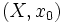$(X,x_0)$ and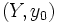$(Y,y_0)$, denoted as$(X,x_0) \vee (Y,y_0)$, is defined as follows:

• As a topological space: It is the quotient of their disjoint union by the identification$\! x_0 \sim y_0$In other words, it is the union of the spaces, identified at the points$x_0$ and$y_0$.
• As a based topological space: It is the above topological space, and the identified point$\! x_0 \sim y_0$ is the chosen basepoint.
• As a based topological space along with inclusions: It is the above based topological space along with the maps from$(X,x_0)$ and$(Y,y_0)$ to it induced by the inclusion in the disjoint union. Both these maps are injective and homeomorphisms to the image. Thus,$(X,x_0)$ and$(Y,y_0)$ both look like subspaces of the wedge sum with the subspace topology.

Note that the homeomorphism type of the wedge sum in general depends on the choice of basepoint for both spaces. The exception is when both spaces are homogeneous spaces. In this case, the homeomorphism type of the wedge sum does not depend on the choice of basepoint. An example is the case of the wedge of two circles.

### For multiple spaces

Suppose$I$ is an indexing set, and for each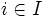$i \in I$, there is a based topological space$(X_i,x_i)$. The wedge sum of these based topological spaces, denoted: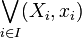$\bigvee_{i \in I} (X_i,x_i)$

is defined as follows:

• As a topological space: It is the quotient of the disjoint union of the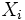$X_i$s by the equivalence relation that identifies all the$x_i$s with each other and makes no other identifications.
• As a based topological space: It is the above topological space, with the basepoint chosen as the single point obtained after identifying all the$x_i$s.
• As a based topological space along with inclusions: It is the above based topological space along with the maps to it from each$(X_i,x_i)$ induced by the inclusion in the disjoint union. All these maps are injective and homeomorphisms to the image. Thus,$(X_i,x_i)$ all look like subspaces of the wedge sum with the subspace topology.

### As a coproduct

The wedge sum is the (unique in the usual sense) coproduct in the category of based topological spaces. In other words, given a bunch of topological spaces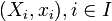$(X_i,x_i), i \in I$, and continuous maps$f_i:(X_i,x_i) \to (Z,z)$ for some based topological space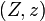$(Z,z)$, there is a unique continuous map$f$ from the wedge sum to$(Z,z)$ such that composing$f$ with the respective inclusion maps gives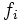$f_i$.

## Facts

### Wedge sum is associative

Since the wedge sum is a coproduct, it is associative in the category of based topological spaces.

However, when the wedge sum is viewed over topological spaces without specification of basepoint, it is not uniquely defined, and hence different ways of associating it may correspond to different interpretations.

For instance, the wedge of three circles has two different interpretations, depending on whether we think of wedging all three circles together, or whether we wedge two circles at one basepoint and then choose a different basepoint in the wedge of two circles to wedge with the third circle.